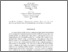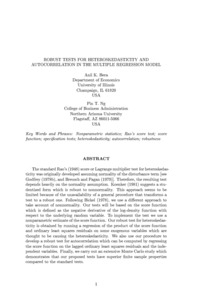# Robust tests for heteroskedasticity and autocorrelation in the multiple regression model: Working paper series--02-05

Bera, Anil K. and Ng, Pin T. (2002) Robust tests for heteroskedasticity and autocorrelation in the multiple regression model: Working paper series--02-05. Working Paper. NAU W.A. Franke College of Business.Preview
Text
Bera_AK_etal_2002_FrankeWPS_02-05(1).pdf

## Abstract

The standard Rao's (1948) score or Lagrange multiplier test for heteroskedasticity was originally developed assuming normality of the disturbance term [see Godfrey (1978b), and Bruesch and Pagan (1979)]. Therefore, the resulting test depends heavily on the normality assumption. Koenker (1981) suggests a studentized for which is robust to nonnormality. This approach seems to be limited because of the unavailability of a general procedure that transforms a test to a robust one. Following Bickel (1978), we use a different approach to take account of nonnormality. Our tests will be based on the score function which is defined as the negative derivitive of the log-density function with respect to the underlying random variable. To implement the test we use a nonparametric estimate of the score function. Our robust test for heteroskedasticity is obtained by running a regression of the product of the score function and ordinary least squares residuals on some exogenous variables which are thought to be causing the heteroskedasticity. We also use our procedure to develop a robust test for autocorrelation which can be computed by regressing the score function on the lagged ordinary least squares residuals and the independent variables. Finally, we carry out an extensive Monte Carlo study which demonstrates that our proposed tests have superior finite sample properties compared to the standard tests.

Item Type: Monograph (Working Paper) Copyright, where appropriate, is held by the author. 02-05 Working paper, Multiple Regression Model, Nonparamteric statistics, Rao's score test, score function, specification tests, heteroskedasticity, autocorrelation, robustness H Social Sciences > HF Commerce Faculty/Staff The W.A. Franke College of Business 19 Jan 2016 19:01 http://openknowledge.nau.edu/id/eprint/1632IR Staff Record View